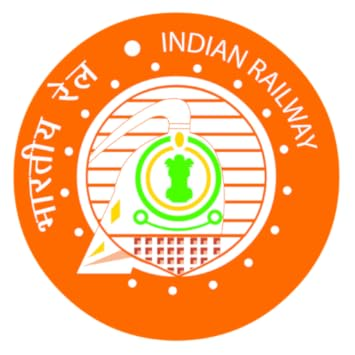Questions Asked in SBI Clerk Prelims Examination (23rd June 2018) 3rd Slot:

1.  12, 12, 36, 180, ?, 11340
2.  6, 7.2, 8.7, 10.8, 14.1, ?
3.  16, 86, 149, 205, ?
4.  7, 14, 40, 103, 227, ?
5.  3, 4, 9, 32, ?, 924

1.  The area of a rectangle and the ratio of the perimeter is 35: 8, which is the length of the rectangle so that the rectangle width is 15 cm?
2.  In an election, there is total 10000 vote. 75% were valid votes & winner candidate win by 2250 vote. The runner-up gets 18% then how many votes do a winner get?
3.  The average age of A, B, and C is 22 years. 3 years ago the average age of b,c is 18 years. Find the age of A, 9 years hence.
4.  The ratio of the boat in still water and speed of the stream is 8:1. Downstream 267.5 in 2.5 hours. What is the upstream?
5.  In an election, there is total 10000 vote. 75% were valid votes & winner candidate win by 2250 vote. The runner-up gets 18% then how many votes do a winner get?
6. √441 – √121 = √x
7.• Data Interpretation based on Pie Chart –
What is the value of half of the difference between the number of students in MBA & MBBS?
How much more students are in MBA as compared to students in B.Ed?
What is the total number of students if taken together in Pharmacy, B.Ed & MBBS?
What is the respective ratio of between Pharmacy students and B.Tech students?
1.1. 8 people sitting in 2 row. A, B, C, D are facing to North & sitting in row 1 while P, Q, R, S were sitting in row 2 facing to South.
2. How many letters in the word BEARING has equal number of letters between as there are in ENGLISH Alphabet Series from left to right only?
3.  In an election, there is total 10000 vote. 75% were valid votes & winner candidate win by 2250 vote. The runner-up gets 18% then how many votes do a winner get?
4. The average age of A, B, and C is 22 years. 3 years ago the average age of b,c is 18 years. Find the age of A, 9 years hence.
5. The ratio of the boat in still water and speed of the stream is 8:1. Downstream 267.5 in 2.5 hours. What is the upstream?

TOP COURSES

Courses offered by UsBANKING

SBI/IBPS/RRB PO,Clerk,SO level ExamsSSC

WBSSC/CHSL/CGL /CPO/MTS etc..RAILWAYS

NTPC/GROUP D/ ALP/JE etc..TEACHING

REET/Super TET/ UTET/CTET/KVS /NVS etc..